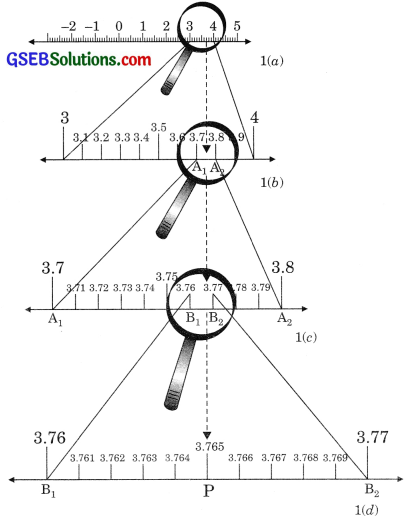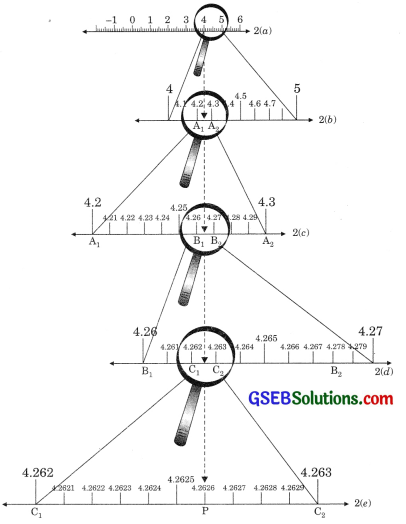# GSEB Solutions Class 9 Maths Chapter 1 Number Systems Ex 1.4

Gujarat Board GSEB Solutions Class 9 Maths Chapter 1 Number Systems Ex 1.4 Textbook Questions and Answers.

## Gujarat Board Textbook Solutions Class 9 Maths Chapter 1 Number Systems Ex 1.4

Question 1.
Visualise 3.765 on the number line using successive magnification.
Solution:
Step 1.
We locate 3.765 on the number line between 3 and 4. To do this we magnify the segment between 3 and 4 and divide this segment in 10 equal parts which shown in figure 1(b).Step 2.
We locate 3.765 on the number line between 3.7 and 3.8 so we magnify the line segment between 3.7 and 3.8 and divide this segment into 10 equal parts which shown in figure 1(c).

Step 3.
Now we observe that 3.765 is between 3.76 and 3.77. Hence again magnify this portion of line segment and divide it into 10 equal parts. The portion of the line segment 3.765 is clearly located on the number line as shown in figure 1(d).Question 2.
Visualise 4.$$\overline { 26 }$$ on the number line, up to 4 places.
Solution:
Step 1.
We locate 4.$$\overline { 26 }$$ up to 4 decimal places i.e., 4.2626 on the number line. We see that 4.2626 is located between 4 and 5 on the number line which is shown in figure 2(a). Divide the segment between 4 and 5 into 10 equal parts and mark each point of division as shown in figure 2(b).

Step 2.
We locate 4.2626 between somewhere of 4.2 and 4.3 and divide this segment into 10 equal parts which is shown in figure 2(c).

Step 3.
Now we magnify the portion between 4.2 and 4.3 by magnifying glass and locate the point 4.2626 in between the point 4.26 and 4.27 which is shown in figure 2(d).

Step 4.
Now locate the point 4.2626 magnifying the line segment between 4.262 and 4.263 by magnifying glass and divide the segment into 10 equal parts and mark the point 4.2626 which is shown in figure 2(e).